# Intro

When we published the GHOST paper on shifting the decision boundary to improve the predictive performance of classification models built on imbalanced datasets, we only considered binary classifiers (e.g. active/inactive, soluble/insoluble, etc.). I was recently asked if the method could be extended to ternary (three-class) classifiers. This post is about doing that.

The code here isn't set up for easy re-use at the moment. It will eventually find its way into the open-source ghostml package once we've had a chance to review and test it more thoroughly.

Aside:the ghostml package is now pip installable: `python -m pip install ghostml` to install it in your environment In order for this to make sense, I think I should start with some explanation of the way I've approached the problem:

## Using thresholds in ternary problems

Things are a bit more complicated here than with binary classifiers. For the binary case we just have a single threshold which determines whether an instance is predicted to be in class 0 or 1. So, assuming that we optimized based on the probability of class 1, we can formulate the decision as:

``````if probabilities >= threshold:
prediction = 1
else:
prediction = 0``````

Before doing any optimization `threshold` is equal to 0.5.

For ternary predictions we have two different decision boundaries and there's no longer a simple threshold; instead the default decision rule can be expressed as:

``prediction = argmax(probabilities)``

i.e., the prediction is the class which has the highest predicted probability.

Aside:the same decision rule can be used for a binary classifier with the default threshold. It's just easier to explain using the threshold of 0.5.
If we want to introduce two thresholds for the ternary classifier, and assuming that we optimize the thresholds for classes 0 and 2, we have to use a more complex decision rule:

``````        if probabilities>=thresholds:
# we might still be in class 2 if the relative probability of that
# is larger than the probability of class 0
if (probabilities-thresholds)>(probabilities-thresholds):
prediction = 2
else:
prediction = 0
elif probabilities>=thresholds:
prediction = 0
else:
prediction = 1``````

## Optimizing thresholds for ternary problems

For the sake of this post let's assume that we're optimizing the thresholds for classes 0 and 2; we could also do 0 and 1, or 1 and 2, the results should still be the same.

In this post I explore two different approaches for optimizing these thresholds.

### Greedy optimization

Here I optimize the two thresholds independently of each other by constructing two binary classification problems and optimizing the thresholds for those problems. Here's the process:

1. Create a binary classification set by setting the training-set `y` values to 1 if the original value is 0 and to 0 otherwise.
2. Use the original `ghostml` approach with that binary classification data and the predicted probabilities of each training point to be 0 in order to set `threshold0`, the threshold for the predicted probability of being 0.
3. Create a binary classification set by setting the training-set `y` values to 1 if the original value is 2 and to 0 otherwise.
4. Use the original `ghostml` approach with that binary classification data and the predicted probabilities of each training point to be 2 in order to set `threshold2`, the threshold for the predicted probability of being 2.

Since the current `ghostml` code doesn't support using balanced accuracy for optimization, I just use kappa for the greedy optimization.

Explore the full grid of possible `(threshold0, threshold2)` pairs and pick the one which produces the optimal Cohen's kappa value. I also try a variant of this which optimizes balanced accuracy instead of Cohen's kappa.

## TL;DR Results summary

Both approaches work well with both simulated data and a couple of datasets from ChEMBL. There doesn't seem to be a large or consistent difference in the quality of the results generated with the two different methods. The greedy optimization approach is, however, quite a bit faster.

Here's the improvement in three scoring metrics (kappa, balanced accuracy, and overall accuracy) when using the greedy optimization procedure on 50 simulated datasets with a 10-80-10 class split; the threshold shift improves both kappa and balanced accuracy on all datasets:And here's the same plot for 20 different random stratified train/tests splits with target CHEMBL205 (carbonic anhydrase II) with activity thresholds chosen to give a 19-72-9 class split. Once again, the threshold shift improves predictive performance:Note: the original version of this notebook and the two CHEMBL data files (file1, file2), are both in github in the older rdkit blog repo.

## Beyond ternary problems

I put some thought into figuring out how to extend this to the general multi-class prediction case, but that turned out to be more difficult than I'd anticipated. If you have suggestions, ideally suggestions accompanied by code, please let me know in the comments!

## Acknowledgements

Many thanks to Ryo Kunimoto and Takayuki Serizawa at Daiichi Sankyo for inspiring and funding the initial part of this work.

And now onto the code and more detailed exploration

```from rdkit import Chem
from rdkit.Chem import rdMolDescriptors
from rdkit.Chem import rdFingerprintGenerator
from rdkit.Chem import PandasTools
# note that you can install ghost using pip: python -m pip install ghostml
import ghostml
import pandas as pd
from sklearn import metrics
import numpy as np

%pylab inline
```
```Populating the interactive namespace from numpy and matplotlib
```

# Code we'll use

```def ternary_rebin(probs,thresholds):
''' returns a list of classifications based on the provided predicted probabilities and thresholds '''
res = []
for prob in probs:
if prob>=thresholds:
# we might still be in class 2 if the relative probability of that
# is larger than the probability of class 0
if (prob-thresholds)>(prob-thresholds):
res.append(2)
else:
res.append(0)
elif prob>=thresholds:
res.append(2)
else:
res.append(1)
return res

def run_ternary_oob_optimization(oob_probs, labels_train, thresholds, ThOpt_metrics = 'Kappa'):
''' does a grid search to optimize the decision thresholds for a ternary problem '''
res = []
tscores = []
for t1 in thresholds:
for t2 in thresholds:
preds = ternary_rebin(oob_probs,(t1,t2))
if ThOpt_metrics == 'Kappa':
tgt = metrics.cohen_kappa_score(labels_train,preds)
elif ThOpt_metrics == 'BalancedAccuracy':
tgt = metrics.balanced_accuracy_score(labels_train,preds)
elif ThOpt_metrics == 'F1':
tgt = metrics.f1_score(labels_train,preds)
tscores.append((np.round(tgt,3),(t1,t2)))
tscores.sort(reverse=True)
thresh = tscores[-1]
return thresh
```
```from sklearn.ensemble import RandomForestClassifier
from sklearn.model_selection import train_test_split

def run_ternary_experiment(X,y,accum,random_state=0):
''' experiment wrapper for the ternary bounds optimization '''
n_classes = max(y)+1
local = {}

# --------------------
# Train - test split
X_train, X_test, y_train, y_test = train_test_split(X, y, test_size = 0.2, stratify = y,
random_state=random_state)

# --------------------
# Train a RF classifier
cls = RandomForestClassifier(n_estimators=500,max_depth=10,oob_score=True,n_jobs=8)
cls.fit(X_train, y_train)

# --------------------
# Calculate the baseline accuracy values
test_preds = cls.predict(X_test)
test_probs = cls.predict_proba(X_test)
kappa = metrics.cohen_kappa_score(y_test,test_preds)
balanced = metrics.balanced_accuracy_score(y_test,test_preds)
accuracy = metrics.accuracy_score(y_test,test_preds)
confusion = metrics.confusion_matrix(y_test,test_preds,labels=list(set(y_test)))
print('original')
print(f'accuracy: {accuracy:.3f}  balanced accuracy: {balanced:.3f}  kappa: {kappa:.3f}')
print(confusion)
local['orig-accuracy'] = accuracy
local['orig-balanced'] = balanced
local['orig-kappa'] = kappa
local['orig-confusion'] = confusion

# --------------------
# optimize the two thresholds individually
thresholds = *(n_classes-1)
for i,clsv in enumerate((0,2)):
d_tform = [1 if y==clsv else 0 for y in y_train]
d_probs = [x[clsv] for x in cls.oob_decision_function_]
thresholds[i] = ghostml.optimize_threshold_from_oob_predictions(d_tform,d_probs,thresholds=np.arange(0.05,1.0,0.05))
local['thresholds'] = thresholds

# calculate the accuracy values for those thresholds:
test_preds = ternary_rebin(test_probs,thresholds)
kappa = metrics.cohen_kappa_score(y_test,test_preds)
balanced = metrics.balanced_accuracy_score(y_test,test_preds)
accuracy = metrics.accuracy_score(y_test,test_preds)
confusion = metrics.confusion_matrix(y_test,test_preds,labels=list(set(y_test)))
print('rebalanced')
print(f'thresholds: {thresholds}')
print(f'accuracy: {accuracy:.3f}  balanced accuracy: {balanced:.3f}  kappa: {kappa:.3f}')
print(confusion)
local['shift-accuracy'] = accuracy
local['shift-balanced'] = balanced
local['shift-kappa'] = kappa
local['shift-confusion'] = confusion

# --------------------
# grid-search optimization of the threshold values based on kappa
thresholds = run_ternary_oob_optimization(cls.oob_decision_function_,y_train,
thresholds=np.arange(0.05,1.00,0.05),
ThOpt_metrics = 'Kappa')
test_preds = ternary_rebin(test_probs,thresholds)
kappa = metrics.cohen_kappa_score(y_test,test_preds)
balanced = metrics.balanced_accuracy_score(y_test,test_preds)
accuracy = metrics.accuracy_score(y_test,test_preds)
confusion = metrics.confusion_matrix(y_test,test_preds,labels=list(set(y_test)))
print('global kappa rebalanced')
print(f'thresholds: {thresholds}')
print(f'accuracy: {accuracy:.3f}  balanced accuracy: {balanced:.3f}  kappa: {kappa:.3f}')
print(confusion)
local['global-k-shift-accuracy'] = accuracy
local['global-k-shift-balanced'] = balanced
local['global-k-shift-kappa'] = kappa
local['global-k-shift-confusion'] = confusion

# --------------------
# grid-search optimization of the threshold values based on the balanced accuracy
thresholds = run_ternary_oob_optimization(cls.oob_decision_function_,y_train,
thresholds=np.arange(0.05,1.00,0.05),
ThOpt_metrics = 'BalancedAccuracy')
test_preds = ternary_rebin(test_probs,thresholds)
kappa = metrics.cohen_kappa_score(y_test,test_preds)
balanced = metrics.balanced_accuracy_score(y_test,test_preds)
accuracy = metrics.accuracy_score(y_test,test_preds)
confusion = metrics.confusion_matrix(y_test,test_preds,labels=list(set(y_test)))
print('global balanced_accuracy rebalanced')
print(f'thresholds: {thresholds}')
print(f'accuracy: {accuracy:.3f}  balanced accuracy: {balanced:.3f}  kappa: {kappa:.3f}')
print(confusion)
local['global-ba-shift-accuracy'] = accuracy
local['global-ba-shift-balanced'] = balanced
local['global-ba-shift-kappa'] = kappa
local['global-ba-shift-confusion'] = confusion

accum.append(local)
```

# Synthetic datasets

I will try out a couple of real datasets below, but I want to start by verifying that the process works with some synthetic datasest. Scikit-learn's make_classification() function makes this really easy.

## Try a 10-80-10 split

I will test this with multiple different forms of imbalance, just to be sure that it generalizes. Let's start with an example where the majority class is in the middle:

```from sklearn.datasets import make_classification

accum_10_80_10 = []

for rep in range(50):
print('--------------')
# Generate a ternary imbalanced classification problem
X, y = make_classification(n_samples=6000, n_features=20,
n_informative=10, n_redundant=0, n_classes=3,
random_state=0xf00d+rep, shuffle=False, weights = [0.1, 0.8, 0.1])
run_ternary_experiment(X,y,accum_10_80_10)
```

Start by comparing the model-performance metrics kappa, balanced accuracy, and accuracy between the model with the greedy threshold shift based on kappa and the model with "default thresholds".

```accum = accum_10_80_10
figsize(9,6)
scatter([x['orig-kappa'] for x in accum],[x['shift-kappa'] for x in accum],label='kappa');
scatter([x['orig-balanced'] for x in accum],[x['shift-balanced'] for x in accum],label='balanced accuracy');
scatter([x['orig-accuracy'] for x in accum],[x['shift-accuracy'] for x in accum],label='accuracy');
plot([.4,1],[.4,1]);
legend();
xlabel('orig')
ylabel('greedy shift');
title('10-80-10');
```The shift improves all three metrics for every dataset.

Now compare the results for using a grid search based on Cohen's kappa to the greedy shift results:

```scatter([x['shift-kappa'] for x in accum],[x['global-k-shift-kappa'] for x in accum],label='kappa');
scatter([x['shift-balanced'] for x in accum],[x['global-k-shift-balanced'] for x in accum],label='balanced accuracy');
scatter([x['shift-accuracy'] for x in accum],[x['global-k-shift-accuracy'] for x in accum],label='accuracy');
plot([.4,1],[.4,1]);
legend();
xlabel('greedy shift')
ylabel('grid-kappa');
title('10-80-10');
```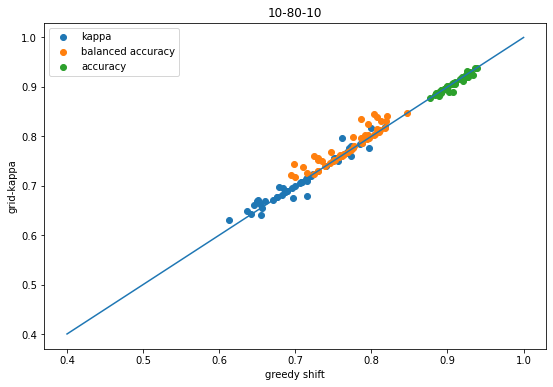Here the changes are reasonably small, but they do tend to slightly favor the results of the grid search.

Finally, do the equivalent plot comparing the result from using balanced accuracy in the grid search to the results from the greedy shift:

```scatter([x['shift-kappa'] for x in accum],[x['global-ba-shift-kappa'] for x in accum],label='kappa');
scatter([x['shift-balanced'] for x in accum],[x['global-ba-shift-balanced'] for x in accum],label='balanced accuracy');
scatter([x['shift-accuracy'] for x in accum],[x['global-ba-shift-accuracy'] for x in accum],label='accuracy');
plot([.4,1],[.4,1]);
legend();
xlabel('greedy shift')
ylabel('grid-balanced');
title('10-80-10');
```That plot makes it look like doing the threshold shifts using balanced accuracy doesn't improve kappa, but it's important to remember that this comparing the balanced accuracy shift vs the kappa shift.

Using balanced accuracy to do the shift instead of kappa does actually help kappa too, as this plot shows:

```scatter([x['orig-kappa'] for x in accum],[x['global-ba-shift-kappa'] for x in accum],label='kappa');
scatter([x['orig-balanced'] for x in accum],[x['global-ba-shift-balanced'] for x in accum],label='balanced accuracy');
scatter([x['orig-accuracy'] for x in accum],[x['global-ba-shift-accuracy'] for x in accum],label='accuracy');
plot([.4,1],[.4,1]);
legend();
xlabel('orig')
ylabel('grid-balanced');
title('10-80-10');
```Still, with these datasets it looks like optimizing the threshold with kappa instead of balanced accuracy is a better idea.

## 0 is the majority class

Now let's make sure that the code doesn't have some "feature" which causes it to only work with the middle class is the majority:

```accum_80_10_10 = []

for rep in range(50):
print('--------------')
# Generate a ternary imbalanced classification problem
X, y = make_classification(n_samples=6000, n_features=20,
n_informative=10, n_redundant=0, n_classes=3,
random_state=0xf00d+rep, shuffle=False, weights = [0.8, 0.1, 0.1])
run_ternary_experiment(X,y,accum_80_10_10)
```
```accum = accum_80_10_10
figsize(9,6)
scatter([x['orig-kappa'] for x in accum],[x['shift-kappa'] for x in accum],label='kappa');
scatter([x['orig-balanced'] for x in accum],[x['shift-balanced'] for x in accum],label='balanced accuracy');
scatter([x['orig-accuracy'] for x in accum],[x['shift-accuracy'] for x in accum],label='accuracy');
plot([.4,1],[.4,1]);
legend();
xlabel('orig')
ylabel('greedy shift');
title('80-10-10');
``````scatter([x['shift-kappa'] for x in accum],[x['global-k-shift-kappa'] for x in accum],label='kappa');
scatter([x['shift-balanced'] for x in accum],[x['global-k-shift-balanced'] for x in accum],label='balanced accuracy');
scatter([x['shift-accuracy'] for x in accum],[x['global-k-shift-accuracy'] for x in accum],label='accuracy');
plot([.4,1],[.4,1]);
legend();
xlabel('greedy shift')
ylabel('grid-kappa');
title('80-10-10');
``````scatter([x['shift-kappa'] for x in accum],[x['global-ba-shift-kappa'] for x in accum],label='kappa');
scatter([x['shift-balanced'] for x in accum],[x['global-ba-shift-balanced'] for x in accum],label='balanced accuracy');
scatter([x['shift-accuracy'] for x in accum],[x['global-ba-shift-accuracy'] for x in accum],label='accuracy');
plot([.4,1],[.4,1]);
legend();
xlabel('greedy shift')
ylabel('grid-balanced');
title('80-10-10');
```Same conclusions as before (good thing!)

## 2 is the majority class

```accum_10_10_80 = []

for rep in range(50):
print('--------------')
# Generate a ternary imbalanced classification problem
X, y = make_classification(n_samples=6000, n_features=20,
n_informative=10, n_redundant=0, n_classes=3,
random_state=0xf00d+rep, shuffle=False, weights = [0.1, 0.1, 0.8])
run_ternary_experiment(X,y,accum_10_10_80)
```
```accum = accum_10_10_80
figsize(9,6)
scatter([x['orig-kappa'] for x in accum],[x['shift-kappa'] for x in accum],label='kappa');
scatter([x['orig-balanced'] for x in accum],[x['shift-balanced'] for x in accum],label='balanced accuracy');
scatter([x['orig-accuracy'] for x in accum],[x['shift-accuracy'] for x in accum],label='accuracy');
plot([.4,1],[.4,1]);
legend();
xlabel('orig')
ylabel('greedy shift');
title('10-10-80');
``````scatter([x['shift-kappa'] for x in accum],[x['global-k-shift-kappa'] for x in accum],label='kappa');
scatter([x['shift-balanced'] for x in accum],[x['global-k-shift-balanced'] for x in accum],label='balanced accuracy');
scatter([x['shift-accuracy'] for x in accum],[x['global-k-shift-accuracy'] for x in accum],label='accuracy');
plot([.4,1],[.4,1]);
legend();
xlabel('greedy shift')
ylabel('grid-kappa');
title('10-10-80');
``````scatter([x['shift-kappa'] for x in accum],[x['global-ba-shift-kappa'] for x in accum],label='kappa');
scatter([x['shift-balanced'] for x in accum],[x['global-ba-shift-balanced'] for x in accum],label='balanced accuracy');
scatter([x['shift-accuracy'] for x in accum],[x['global-ba-shift-accuracy'] for x in accum],label='accuracy');
plot([.4,1],[.4,1]);
legend();
xlabel('greedy shift')
ylabel('grid-balanced');
title('10-10-80');
```Same conclusions as before (good thing!)

# Some ChEMBL datasets

Let's just be sure that this approach works with bioactivity data too. I don't think it's necessary do a comprehensive evaluation here, but I want to show a couple of examples. I didn't cherry pick these.

## CHEMBL205: Carbonic Anhydrase II

```data = pd.read_csv('../data/target_CHEMBL205.csv.gz')
data['pKi'] = [-math.log10(x*1e-9) for x in data['standard_value']]
```
compound_chembl_id canonical_smiles standard_value standard_units standard_relation standard_type year ROMol pKi
0 CHEMBL1054 NS(=O)(=O)c1cc2c(cc1Cl)NC(C(Cl)Cl)NS2(=O)=O 91.0 nM = Ki 2009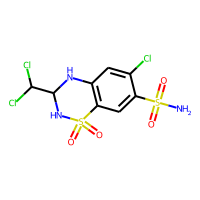7.040959
1 CHEMBL1055 NS(=O)(=O)c1cc(C2(O)NC(=O)c3ccccc32)ccc1Cl 138.0 nM = Ki 2009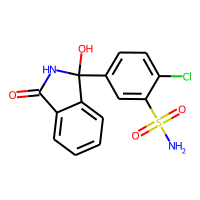6.860121
2 CHEMBL1060 O=P([O-])([O-])O.[Na+].[Na+] 13200000.0 nM = Ki 2004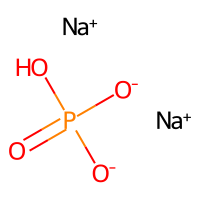1.879426
3 CHEMBL106848 NS(=O)(=O)c1ccc(SCCO)cc1 21.0 nM = Ki 2013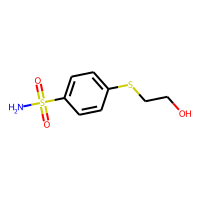7.677781
4 CHEMBL107217 CCN(CC)C(=S)[S-].[Na+] 3100.0 nM = Ki 2009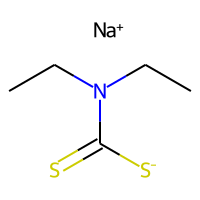5.508638

Pick two pKi values for binning

```def binner(act,bins=(5,8.5)):
for i,bin in enumerate(bins):
if act<=bin:
return i
return len(bins)
data['activity'] = [binner(x) for x in data.pKi]
data.groupby('activity').describe()
```
standard_value year pKi
count mean std min 25% 50% 75% max count mean ... 75% max count mean std min 25% 50% 75% max
activity
0 968.0 1.242009e+18 3.864224e+19 10000.000 10000.0000 50000.0 196700.000 1.202264e+21 968.0 2012.994835 ... 2016.0 2020.0 968.0 4.069107 1.200449 -12.080000 3.706216 4.301030 5.000000 5.00000
1 3582.0 7.292523e+02 1.778519e+03 3.200 13.5000 73.4 417.750 9.900000e+03 3582.0 2013.261307 ... 2017.0 2020.0 3582.0 7.050231 0.915651 5.004365 6.379084 7.134306 7.869666 8.49485
2 427.0 1.309327e+00 8.709364e-01 0.008 0.6355 1.0 2.035 3.100000e+00 427.0 2014.962529 ... 2017.0 2020.0 427.0 9.050659 0.500779 8.508638 8.691437 9.000000 9.196895 11.09691

3 rows × 24 columns

Ok, that's imbalanced :-)

Generate fingerprints:

```from rdkit.Chem import SaltRemover
sr = SaltRemover.SaltRemover()
stripped = [sr.StripMol(m) for m in data.ROMol]
fps = [fpgen.GetFingerprint(m) for m in stripped]
```

And now run the experiment with 20 random splits:

```accum_chembl205 = []
for i in range(20):
run_ternary_experiment(fps,data.activity,accum_chembl205,random_state=0xf00d+i)
```
```accum = accum_chembl205
figsize(9,6)
scatter([x['orig-kappa'] for x in accum],[x['shift-kappa'] for x in accum],label='kappa');
scatter([x['orig-balanced'] for x in accum],[x['shift-balanced'] for x in accum],label='balanced accuracy');
scatter([x['orig-accuracy'] for x in accum],[x['shift-accuracy'] for x in accum],label='accuracy');
plot([.4,1],[.4,1]);
legend();
xlabel('orig')
ylabel('greedy shift');
title('CHEMBL205');
``````scatter([x['shift-kappa'] for x in accum],[x['global-k-shift-kappa'] for x in accum],label='kappa');
scatter([x['shift-balanced'] for x in accum],[x['global-k-shift-balanced'] for x in accum],label='balanced accuracy');
scatter([x['shift-accuracy'] for x in accum],[x['global-k-shift-accuracy'] for x in accum],label='accuracy');
plot([.4,1],[.4,1]);
legend();
xlabel('greedy shift')
ylabel('grid-kappa');
title('CHEMBL205');
``````scatter([x['shift-kappa'] for x in accum],[x['global-ba-shift-kappa'] for x in accum],label='kappa');
scatter([x['shift-balanced'] for x in accum],[x['global-ba-shift-balanced'] for x in accum],label='balanced accuracy');
scatter([x['shift-accuracy'] for x in accum],[x['global-ba-shift-accuracy'] for x in accum],label='accuracy');
plot([.4,1],[.4,1]);
legend();
xlabel('greedy shift')
ylabel('grid-balanced');
title('CHEMBL205');
```We see the same behavior as before: shifting the descision thresholds using either the greedy approach or grid-based approach improves prediction accuracy over the default decision thresholds.

## CHEMBL217: Dopamine D2

```data = pd.read_csv('../data/target_CHEMBL217.csv.gz')
data['pKi'] = [-math.log10(x*1e-9) for x in data['standard_value']]
def binner(act,bins=(5,8)):
for i,bin in enumerate(bins):
if act<=bin:
return i
return len(bins)
data['activity'] = [binner(x) for x in data.pKi]
data.groupby('activity').describe()
```
standard_value year pKi
count mean std min 25% 50% 75% max count mean ... 75% max count mean std min 25% 50% 75% max
activity
0 356.0 143415.189354 781194.668326 10000.000 10000.0000 10000.00 24234.5 10000000.00 356.0 2011.679775 ... 2017.0 2019.0 356.0 4.672916 0.581865 2.000000 4.615626 5.000000 5.000000 5.000000
1 4014.0 830.546163 1471.610125 10.000 63.1875 238.51 931.0 9906.00 4014.0 2011.100648 ... 2015.0 2020.0 4014.0 6.620074 0.724919 5.004102 6.031050 6.622494 7.199370 8.000000
2 607.0 3.715942 2.786155 0.027 1.2150 3.00 5.9 9.86 607.0 2011.957166 ... 2016.0 2019.0 607.0 8.614671 0.475862 8.006123 8.229148 8.522879 8.915457 10.568636

3 rows × 24 columns

```from rdkit.Chem import SaltRemover
sr = SaltRemover.SaltRemover()
stripped = [sr.StripMol(m) for m in data.ROMol]
fps = [fpgen.GetFingerprint(m) for m in stripped]
```
```accum_chembl217 = []
for i in range(20):
run_ternary_experiment(fps,data.activity,accum_chembl217,random_state=0xf00d+i)
```
```accum = accum_chembl217
figsize(9,6)
scatter([x['orig-kappa'] for x in accum],[x['shift-kappa'] for x in accum],label='kappa');
scatter([x['orig-balanced'] for x in accum],[x['shift-balanced'] for x in accum],label='balanced accuracy');
scatter([x['orig-accuracy'] for x in accum],[x['shift-accuracy'] for x in accum],label='accuracy');
plot([.2,1],[.2,1]);
legend();
xlabel('orig')
ylabel('greedy shift');
title('CHEMBL217');
```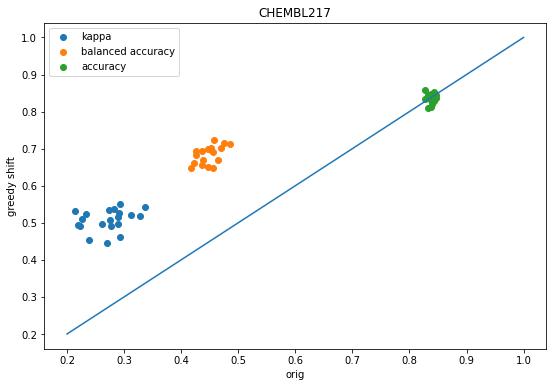```scatter([x['shift-kappa'] for x in accum],[x['global-k-shift-kappa'] for x in accum],label='kappa');
scatter([x['shift-balanced'] for x in accum],[x['global-k-shift-balanced'] for x in accum],label='balanced accuracy');
scatter([x['shift-accuracy'] for x in accum],[x['global-k-shift-accuracy'] for x in accum],label='accuracy');
plot([.4,1],[.4,1]);
legend();
xlabel('greedy shift')
ylabel('grid-kappa');
title('CHEMBL217');
``````scatter([x['shift-kappa'] for x in accum],[x['global-ba-shift-kappa'] for x in accum],label='kappa');
scatter([x['shift-balanced'] for x in accum],[x['global-ba-shift-balanced'] for x in accum],label='balanced accuracy');
scatter([x['shift-accuracy'] for x in accum],[x['global-ba-shift-accuracy'] for x in accum],label='accuracy');
plot([.4,1],[.4,1]);
legend();
xlabel('greedy shift')
ylabel('grid-balanced');
title('CHEMBL217');
```Again, the same conclusions hold here.